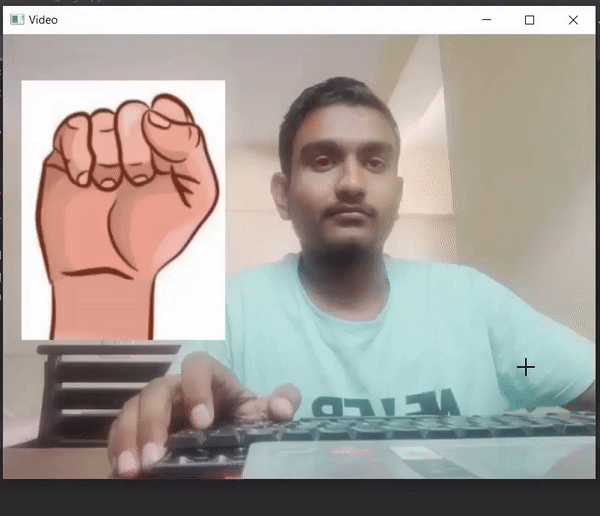# Creating a Finger Counter Using Computer Vision and OpenCv in Python

• Last Updated : 25 Apr, 2022

In this article, we are going to create a finger counter using Computer Vision, OpenCv in Python

## Required Libraries:

• OpenCV: OpenCV is a widely used library for image processing.
• cvzone: It is a computer vision package that makes it easy to run Image processing and AI functions

To install the Cvzone, run this command into terminal:

`pip install cvzone`

## Stepwise Implementation:

Step 1: Import required libraries.

## Python3

 `# import libraries and required classes` `import` `cv2` `from` `cvzone.HandTrackingModule ``import` `HandDetector`

Step 2: Declare the HandDetector and open the default camera to capture the hand.

## Python3

 `# declaring HandDetector with` `# some basic requirements` `detector ``=` `HandDetector(maxHands``=``1``,` `                        ``detectionCon``=``0.8``)`   `# it detect only one hand from` `# video with 0.8 detection confidence` `video ``=` `cv2.VideoCapture(``0``)`

Step 3: Count the number of fingers

Capture the frames continuously and detect the hand from the frame then detect how many fingers are up and count them. On the counter make appropriate conditions and place an image of fingers.

## Python3

 `while` `True``:` `      ``# Capture frame-by-frame ` `    ``_, img ``=` `video.read()` `    ``img ``=` `cv2.flip(img, ``1``)` `    `  `    ``# Find the hand with help of detector` `    ``hand ``=` `detector.findHands(img, draw``=``False``)` `    `  `    ``# Here we take img by default if no hand found` `    ``fing ``=` `cv2.imread(``"Put image path with 0 fingures up"``)` `    ``if` `hand:` `      `  `          ``# Taking the landmarks of hand` `        ``lmlist ``=` `hand[``0``] ` `        ``if` `lmlist:` `          `  `              ``# Find how many fingers are up` `            ``# This function return list` `            ``fingerup ``=` `detector.fingersUp(lmlist)  ` `            `  `            ``# Change image based on ` `            ``# different-different conditions` `            ``if` `fingerup ``=``=` `[``0``, ``1``, ``0``, ``0``, ``0``]:` `                ``fing ``=` `cv2.imread("Put image path of ``1``\` `                ``fingures up")` `            ``if` `fingerup ``=``=` `[``0``, ``1``, ``1``, ``0``, ``0``]:` `                ``fing ``=` `cv2.imread("Put image path of ``2``\` `                ``fingures up")` `            ``if` `fingerup ``=``=` `[``0``, ``1``, ``1``, ``1``, ``0``]:` `                ``fing ``=` `cv2.imread("Put image path of\` `                ``3` `fingures up")` `            ``if` `fingerup ``=``=` `[``0``, ``1``, ``1``, ``1``, ``1``]:` `                ``fing ``=` `cv2.imread("sPut image path \` `                ``of ``4` `fingures up")` `            ``if` `fingerup ``=``=` `[``1``, ``1``, ``1``, ``1``, ``1``]:` `                ``fing ``=` `cv2.imread("Put image path \` `                ``of ``4` `fingures ``and` `thumbs up")` `    ``# Resize the image` `    ``fing ``=` `cv2.resize(fing, (``220``, ``280``))` `    `  `    ``# Place the image in main frame` `    ``img[``50``:``330``, ``20``:``240``] ``=` `fing` `    `  `    ``# Display the resulting frame` `    ``cv2.imshow(``"Video"``, img)`

How actually detector detects the fingers up or down.

• It returns the list of five elements and each element depends on the finger’s condition.
• The list order like [ thumb, index finger, middle finger, ring finger, pinky/little finger ]
• If any of the fingers are up, it returns 1 for that particular index value else returns 0.
• And after making the list it will return by the function.

Step 4: Terminate the loop

## Python3

 `# Enter key 'q' to break the loop` `if` `cv2.waitKey(``1``) & ``0xFF` `=``=` `ord``(``'q'``):` `    ``break` `    `  `# When everything done, release` `# the capture and destroy the windows` `video.release()` `cv2.destroyAllWindows()`

## Python3

 `import` `cv2` `from` `cvzone.HandTrackingModule ``import` `HandDetector`   `detector ``=` `HandDetector(maxHands``=``1``, detectionCon``=``0.8``)` `video ``=` `cv2.VideoCapture(``1``)`   `while` `True``:` `    ``_, img ``=` `video.read()` `    ``img ``=` `cv2.flip(img, ``1``)` `    ``hand ``=` `detector.findHands(img, draw``=``False``)` `    ``fing ``=` `cv2.imread(``"Put image path with 0 fingures up"``)` `    ``if` `hand:` `        ``lmlist ``=` `hand[``0``]` `        ``if` `lmlist:` `            ``fingerup ``=` `detector.fingersUp(lmlist)` `            ``if` `fingerup ``=``=` `[``0``, ``1``, ``0``, ``0``, ``0``]:` `                ``fing ``=` `cv2.imread("Put image \` `                ``path of ``1` `fingures up")` `            ``if` `fingerup ``=``=` `[``0``, ``1``, ``1``, ``0``, ``0``]:` `                ``fing ``=` `cv2.imread("Put image \` `                ``path of ``2` `fingures up")` `            ``if` `fingerup ``=``=` `[``0``, ``1``, ``1``, ``1``, ``0``]:` `                ``fing ``=` `cv2.imread("Put image \` `                ``path of ``3` `fingures up")` `            ``if` `fingerup ``=``=` `[``0``, ``1``, ``1``, ``1``, ``1``]:` `                ``fing ``=` `cv2.imread("Put image \` `                ``path of ``4` `fingures up")` `            ``if` `fingerup ``=``=` `[``1``, ``1``, ``1``, ``1``, ``1``]:` `                ``fing ``=` `cv2.imread("Put image \` `                ``path of ``4` `fingures ``and` `thumbs up")` `    ``fing ``=` `cv2.resize(fing, (``220``, ``280``))` `    ``img[``50``:``330``, ``20``:``240``] ``=` `fing` `    ``cv2.imshow(``"Video"``, img)` `    `  `    ``if` `cv2.waitKey(``1``) & ``0xFF` `=``=` `ord``(``'q'``):` `        ``break` `        `  `video.release()` `cv2.destroyAllWindows()`

Output :My Personal Notes arrow_drop_up
Recommended Articles
Page :